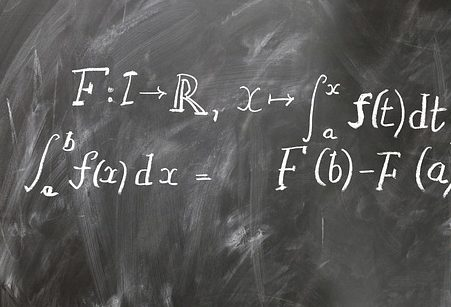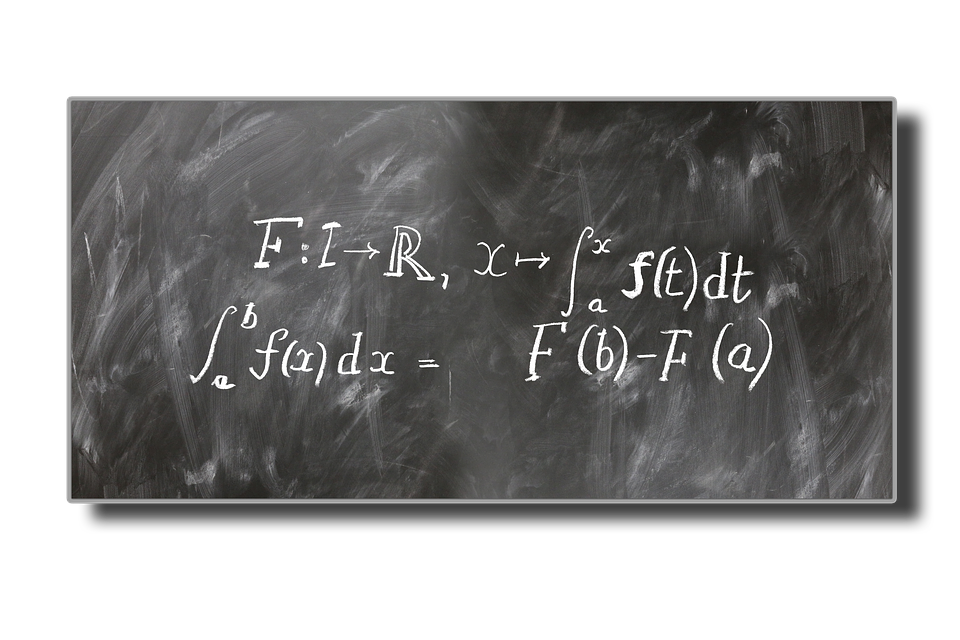# How To Calculate Hyperbolic Derivatives

Hyperbolic derivatives may sound scary, but they are easy. In fact, their properties are among the most elegantly simple in all of mathematics.A hyperbolic derivative is a derivate of one of the hyperbolic functions, which are functions that utilize the exponential function (ex) to simplify otherwise complex calculations. Common uses for hyperbolic functions include representing the length of arcs such as those formed by the cables of a suspension bridge, or the shape of the Gateway Arch in St. Louis, Missouri.

As derivatives calculate the rate of change of a function (such as deriving a function for a car’s acceleration given a function of its velocity over a variable of time), calculating a hyperbolic derivative means calculating the rate of change of a hyperbolic function. This definition is especially useful for understanding hyperbolic integrals, which are helpful in arc length calculations and other applications of mathematics and engineering.

If some of these terms are unfamiliar (or outright intimidating), don’t worry! We’ll give a quick review of some of the basics before diving into the actual derivations.

## A Review ​Of Trigonometry

Hyperbolic functions are closely related to trigonometry, as the functions that they describe relate to a hyperbola in the same way that trigonometric functions relate to a unit circle. But what exactly is this relationship, or even the “unit circle” for that matter? Is there a “unit hyperbola?”

Trigonometry helps describe the “unit circle” represented by function x2+y2=1. On a two-dimensional graph, this circle has its center at the origin (point (0,0)) with a radius of one unit. Trigonometry is the study of the dimensions within this circle, where special functions provide the height and width of the circle’s radius at a certain angle. The “certain angle” part is especially important.

This concept can be challenging to visualize internally, but consider an easy example: Suppose that the unit circle’s “certain angle” starts on the x-axis at zero degrees. In other words, if we position a line within the unit circle directly on the x-axis, it will be at zero degrees relative to the rest of the circle. Rotating it about the origin towards the positive y-axis will increase this angle in the positive direction while turning it towards the negative y-axis will decrease this angle in the negative direction.

If we leave our imaginary line on the x-axis, what are the “components” of its height and width? Well, at zero degrees (or the x-axis), its width is its length. So, if our imaginary line has the same radius as the unit circle (one unit), then we could say that the “width” component of our circle is also one unit.

However, what about our height component? Where do we start measuring? We measure the height component of our line from the x-axis, just as we measured its width from the y-axis. So, since our line is lying directly on the x-axis, its height component must be zero.

As we rotate our imaginary line inside the unit circle, the height and width components of our imaginary line will change with the angle of rotation. Laying the line directly on the positive y-axis, for example, will have height and width components of one and zero units, respectively. But what about points in between?

Thankfully, the height and width components of our line are conveniently described using basic trigonometric functions. Here, the height component is the function sin⁡(x) (pronounced “sine of x”), and the width component is the function cos⁡(x) (pronounced “cosine of x”). Using zero as a value for “x” in both of these equations will yield our original width and height of one and zero, respectively.

We’ll come back to this subject later once we explore their similarities with the hyperbolic functions. Until then, we also have to review the all-important exponential function.

## A Review ​Of ​The Exponential Function (ex)

The exponential function is one of the single most essential functions in all of mathematics. We won’t describe its full capabilities and applications here, but for now, understand that it adequately describes the growth of just about anything in the natural world: plant growth, radioactive decay and numerous other phenomena are all accurately quantified using the exponential function.

The exponential function is represented by y=ex, where e=2.71828…. On a graph, this forms a hyperbola that grows at an exponential rate. e, also known as “Euler’s number,” just-so-happens to be a near-universal ratio of growth.

This function is (hopefully) interesting and all, but how does it relate to hyperbolic functions?

## The Hyperbolic Functions: Combining Trigonometry ​And ​The Exponential FunctionImage Source : Pixabay

Hyperbolic functions describe the hyperbola of the exponential function in a similar way that trigonometric functions describe the unit circle. The names of the basic “height” and “width” functions are remarkably similar to those from trigonometry!

Where trigonometric functions include sin x and cos⁡(x), hyperbolic functions include sinh(x) (pronounced “cinch of x”) and koshx (pronounced “kosh of x” or “kosh” rhymes with “gosh”). These are defined using the exponential function such that:

sinh x =ex-e-x2   and   x =ex+e-x2

Note the subtle difference between the functions: The numerator of the sinh(x) function is ex-e-x, while that of the koshx function is ex+e-x.

The names and functions of the hyperbolic functions aren’t their only similarities to trigonometry; other hyperbolic functions and identities are, in fact, remarkably similar to their counterparts in trigonometry.

One example of this similarity is the “double angle” identity, which in trigonometry is sin 2x =2sin x cos x . What happens if we work backward and replace sin x and cos⁡(x) with their hyperbolic cousins? Something interesting happens:

2sinh x x =2ex-e-x2ex+e-x2=e2x-e-2×2=sinh 2x

The identity is the same as it is in trigonometry! Other trigonometric parallels are also possible but are somewhat beyond the scope of this guide.

For now, we’ve defined the two most basic hyperbolic functions— sinh(x) and koshx. At this point, you’ve probably determined that finding a hyperbolic derivative involves finding the derivative of one of these (or one of the other) functions.

Before actually calculating hyperbolic derivatives, however, we’ll have a quick review on regular derivatives. This review will be especially useful, as the exponential function has some very unique properties when it comes to derivation.

## A Review ​Of Derivatives

Again, a derivative of a function is its rate of change with a variable. Returning to the car analogy, we might be able to describe a car’s distance traveled as a function of time. In other words, we could have a function that says our car moves a distance of “x” over time “t” such that x=50t.

If we assign “x” to have its unit in meters and “t” to have its unit in seconds, we could say that our car will cover a distance of 50 meters in one second (which, by the way, is very fast—about 112 miles per hour!).

We might be curious to know, then, how fast the car is going. Intuition tells us that if the car travels 50 meters in one second, it must have a speed of 50 meters per second. Just by going through this mental exercise, you’ve calculated a derivative!

The exact theory of derivation is well beyond the scope of this guide. For now, remember that a derivative of a function describes that functions rate of change.

When solving a derivative algebraically, one of the most powerful rules to remember is the “power rule.” Here, “power” refers to whatever power a function’s variable is raised to. For example, the function y=x2 has the variable “x” raised to a power of two.

What would be the derivative of such a function? Or, in other words, what would be its rate of change as we change the value of “x?” The power rule offers a convenient solution, stating that the derivative of xn=n*xn-1. So, in the case of y=x2, dydx=2x (here, dydx means “the derivative of function ‘y’ by variable ‘x’”).

What if we try that on our car’s position equation from earlier? A quick calculation shows that x’=1*50t1-1=50, which is exactly what our intuition told us.

This rule works for most algebraic equations, but some functions have special derivation rules. One of these is the exponential function from earlier, whose derivative is – wait for it – itself! Formally stated, this relationship takes the form dydxenx=nenx. Take special care to remember any constant in front of the variable “x!”

As you might be able to imagine, this relationship is especially helpful for calculating hyperbolic derivatives—which we can now finally do.

## Calculating Hyperbolic DerivativesImage Source : Pixabay

Now that we have a solid background, we can move forward to deriving our essential hyperbolic functions. With the knowledge from the previous sections, the derivations should be reasonably straightforward.

For calculating the derivative of sinh x , we derivate its value of ex-e-x2. Using the rules described in the previous section, this yields an exciting result: ex+e-x2. This value looks a lot like x because it is! Similarly, deriving x will produce the value of sinh x .

## Conclusion

It appears that the derivatives of the two essential hyperbolic functions sinh x and x are, in fact, each other. Remembering the parallels between hyperbolic and trigonometric identities, one can easily derive hyperbolic functions such as tanh x , where tanh x =sinh⁡(x)kosh(x) just as tan x =sin⁡(x)cos(x). Can you find its derivative?

–>